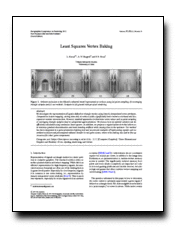# "Least Squares Vertex Baking"We investigate the representation of signals defined on triangle meshes using linearly interpolated vertex attributes. Compared to texture mapping, storing data only at vertices yields significantly lower memory overhead and less expensive runtime reconstruction. However, standard approaches to determine vertex values such as point sampling or averaging triangle samples lead to suboptimal approximations. We discuss how an optimal solution can be efficiently calculated using continuous least-squares. In addition, we propose a regularization term that allows us to minimize gradient discontinuities and mach banding artifacts while staying close to the optimum. Our method has been integrated in a game production lighting tool and we present examples of representing signals such as ambient occlusion and precomputed radiance transfer in real game scenes, where vertex baking was used to free up resources for other game components. L. Kavan, A.W. Bargteil, P.-P. Sloan "Least Squares Vertex Baking." In Computer Graphics Forum (EGSR 2011), June 2011, Vol 30, No 4. Download PDF           BibTex